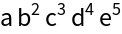Function Repository Resource:

FoldIndexed

Perform a folding operation while also parsing the index as the third argument

Contributed by: Sander Huisman
 ResourceFunction["FoldIndexed"][f,x,{a,b,…}] gives the last element of FoldListIndexed[f,x,{a,b,…}]. ResourceFunction["FoldIndexed"][f,{a,b,c,…}] is equivalent to ResourceFunction["FoldIndexed"][f,First[a],Rest[a]]. ResourceFunction["FoldIndexed"][f] represents an operator form of ResourceFunction["FoldIndexed"] that can be applied to expressions.

Details and Options

ResourceFunction["FoldIndexed"][f][list] is equivalent to ResourceFunction["FoldIndexed"][f,list].
ResourceFunction["FoldIndexed"][f][x,list] is equivalent to ResourceFunction["FoldIndexed"][f,x,list].

Examples

Basic Examples

Basic usage:

 In:=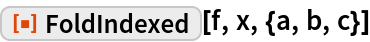Out=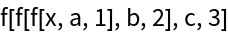Scope

Without an initializer, the first element of list is the initializer:

 In:=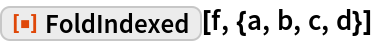Out=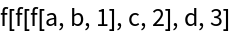Make an operator:

 In:=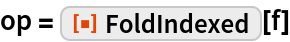Out=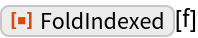Feed a single list to the operator:

 In:=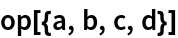Out=Feed an initializer and a list to the operator:

 In:=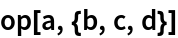Out=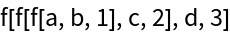Applications

Form a continued fraction with increasing power:

 In:=Out=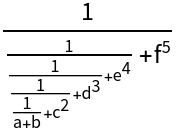Form a continued fraction with an increasing numerator:

 In:=Out=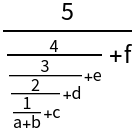Form the following product: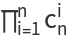:

 In:=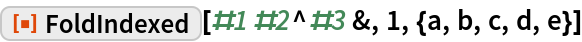Out=